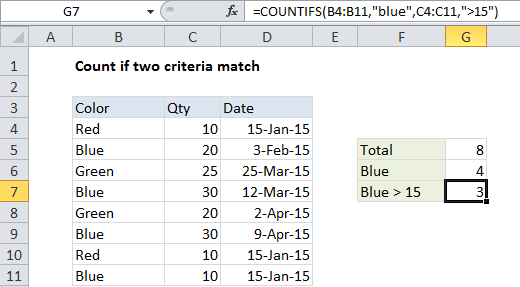## Excel Office

Excel How Tos, Tutorials, Tips & Tricks, Shortcuts

# Count if two criteria match in Excel

This tutorial shows how to Count if two criteria match in Excel using the example below;

## Formula

`=COUNTIFS(range1,critera1,range2,critera2)`## Explanation

If you want to count rows where two (or more) criteria match, you can use a formula based on the COUNTIFS function.

In the example shown, we want to count the number of orders with a color of “blue” and a quantity > 15. The formula we have in cell G7 is:

`=COUNTIFS(B4:B11,"blue",C4:C11,">15")`

### How this formula works

The COUNTIFS function takes multiple criteria in pairs — each pair contains one range and the associated criteria for that range. To generate a count, all conditions must match. To add more conditions, just add another range / criteria pair.

Also See:   Check if range contains a value not in another range in Excel

### SUMPRODUCT alternative

You can also use the SUMPRODUCT function to count rows that match multiple conditions. the equivalent formula is:

`=SUMPRODUCT((B4:B11="Blue")*(C4:C11>15))`

SUMPRODUCT is more powerful and flexible than COUNTIFS, and it works with all Excel versions, but it is not as fast with larger sets of data.

### Pivot table alternative

If you need to summarize  number of criteria combinations in a larger data set, you should consider pivot tables. Pivot tables are a fast and flexible reporting tool that can summarize data in many different ways.

Also See:   Sum bottom n values in Excel